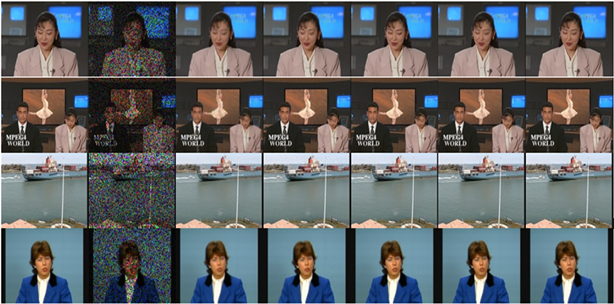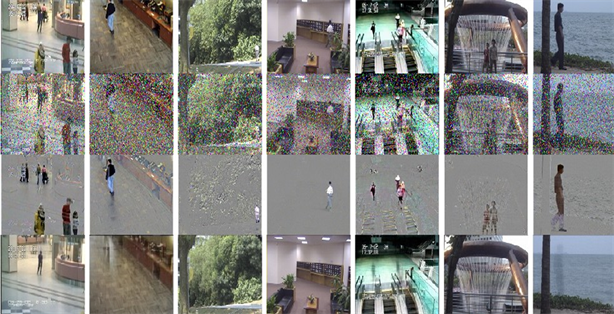#### 期刊菜单

Image and Video Recovery Based on Low-Rank High-Order Tensor Approximation
DOI: 10.12677/AIRR.2022.112009, PDF, HTML, XML, 下载: 52  浏览: 101

Abstract: Image and video recovery is a basic but key task in computer vision and has been widely studied in recent years. However, existing methods have inevitable disadvantages: some require pre-defined rank, while others cannot handle high-order data. In order to overcome these shortcomings, this paper uses the low-rank high-order tensor approximation method to realize color video recovery in mixed noise environment. Firstly, a high-order tensor algebraic framework is established. Based on the framework, a new low-rank high-order tensor approximation (LRHA) method is proposed by designing a proximal operator to recover potential low-rank parts from highly contaminated tensor data, so as to complete the task of image and video restoration. And the corresponding algorithm was designed. Experimental results of image and video restoration tasks show that LRHA has advantages in dealing with corresponding problems.

1. 引言

2. 符号和数学框架

2.1. 符号

2.2. DFT共轭性质

${F}_{n}\cdot circ\left(a\right)\cdot {F}_{n}^{-1}=diag\left( a ¯ \right)$

$\left\{\begin{array}{l}{\stackrel{¯}{A}}^{\left(1,1,\cdots ,1\right)}\in {ℝ}^{{n}_{1}×{n}_{2}}\hfill \\ conj\left({\stackrel{¯}{A}}^{\left({n}_{3}-{i}_{3}+2,1,\cdots ,1\right)}\right)={\stackrel{¯}{A}}^{\left({i}_{3},1,\cdots ,1\right)},\hfill \\ conj\left({\stackrel{¯}{A}}^{\left({{n}^{\prime }}_{3},{n}_{4}-{i}_{4}+2,1,\cdots ,1\right)}\right)={\stackrel{¯}{A}}^{\left({{i}^{″}}_{3},{i}_{4},1,\cdots ,1\right)},\hfill \\ \text{ }\text{ }\text{ }⋮\hfill \\ conj\left({\stackrel{¯}{A}}^{\left({{n}^{\prime }}_{3},{{n}^{\prime }}_{4},1,\cdots ,{n}_{p}-{i}_{p}+2\right)}\right)={\stackrel{¯}{A}}^{\left({{i}^{″}}_{3},{{i}^{″}}_{4},\cdots ,{{i}^{″}}_{p-1},{i}_{p}\right)},\hfill \end{array}$ (2.1)

${{n}^{\prime }}_{j}=\left\{\begin{array}{l}1,{i}_{j}=1,\hfill \\ {n}_{j}-{i}_{j}+2,{i}_{j}\ne 1.\hfill \end{array}$${{i}^{″}}_{k}=1,\cdots ,{n}_{k}$${i}_{l}=2,\cdots ,⌊\frac{{n}_{k}+1}{2}⌋$$l=3,\cdots ,p$$j,k=3,\cdots ,p-1$

${\stackrel{¯}{A}}^{p}=\left({F}_{{n}_{p}}\otimes {F}_{{n}_{p-1}}\otimes \cdots \otimes {F}_{{n}_{3}}\otimes {I}_{{n}_{1}}\right){\stackrel{˜}{A}}^{p}\left({F}_{{n}_{p}}^{*}\otimes {F}_{{n}_{p-1}}^{*}\otimes \cdots \otimes {F}_{{n}_{3}}^{*}\otimes {I}_{{n}_{2}}\right)$

${\stackrel{˜}{A}}^{p}=\left[\begin{array}{cccc}{\stackrel{˜}{A}}^{p-1\text{ }\left(1\right)}& {\stackrel{˜}{A}}^{p-1\text{ }\left({n}_{p}\right)}& \cdots & {\stackrel{˜}{A}}^{p-1\text{ }\left(2\right)}\\ {\stackrel{˜}{A}}^{p-1\text{ }\left(2\right)}& {\stackrel{˜}{A}}^{p-1\text{ }\left(1\right)}& \cdots & {\stackrel{˜}{A}}^{p-1\text{ }\left(3\right)}\\ ⋮& ⋮& \ddots & ⋮\\ {\stackrel{˜}{A}}^{p-1\text{ }\left({n}_{p}\right)}& {\stackrel{˜}{A}}^{p-1\text{ }\left({n}_{p-1}\right)}& \cdots & {\stackrel{˜}{A}}^{p-1\text{ }\left(1\right)}\end{array}\right]$

${\stackrel{˜}{A}}^{p-1\text{ }\left({i}_{p}\right)}\in {ℝ}^{{n}_{1}{n}_{3}{n}_{4}\cdots {n}_{p-1}×{n}_{2}{n}_{3}{n}_{4}\cdots {n}_{p-1}}$

${\stackrel{˜}{A}}^{p-1\text{ }\left({i}_{p}\right)}=\left[\begin{array}{cccc}{\stackrel{˜}{A}}^{p-2\text{ }\left(1,{i}_{p}\right)}& {\stackrel{˜}{A}}^{p-2\text{ }\left({n}_{p-1},{i}_{p}\right)}& \cdots & {\stackrel{˜}{A}}^{p-2\text{ }\left(2,{i}_{p}\right)}\\ {\stackrel{˜}{A}}^{p-2\text{ }\left(2,{i}_{p}\right)}& {\stackrel{˜}{A}}^{p-2\text{ }\left(1,{i}_{p}\right)}& \cdots & {\stackrel{˜}{A}}^{p-2\text{ }\left(3,{i}_{p}\right)}\\ ⋮& ⋮& \ddots & ⋮\\ {\stackrel{˜}{A}}^{p-2\text{ }\left({n}_{p-1},{i}_{p}\right)}& {\stackrel{˜}{A}}^{p-2\text{ }\left({n}_{p-1}-1,{i}_{p}\right)}& \cdots & {\stackrel{˜}{A}}^{p-2\text{ }\left(1,{i}_{p}\right)}\end{array}\right]$

2.3. 代数框架

$unfold\left({\mathcal{A}}^{p}\right)=\left[\begin{array}{c}{\mathcal{A}}^{p-1\text{ }\left(1\right)}\\ {\mathcal{A}}^{p-1\text{ }\left(2\right)}\\ ⋮\\ {\mathcal{A}}^{p-1\text{ }\left({n}_{p}\right)}\end{array}\right]$$fold\left(unfold\left({\mathcal{A}}^{p}\right)\right)={\mathcal{A}}^{p}$

$unfold$ 算子将 ${\mathcal{A}}^{p}$ 按照第p维度展开为 ${n}_{p}$ 个大小为 ${n}_{1}×{n}_{2}×\cdots ×{n}_{p-1}$ 的张量且 $fold$ 是它的逆算子。

$tcirc\left({\mathcal{A}}^{p}\right)={\stackrel{˜}{A}}^{p}$$tunfold\left({\mathcal{A}}^{p}\right)={\stackrel{^}{A}}^{p}=\left[\begin{array}{c}{\stackrel{^}{A}}^{p-1\text{ }\left(1\right)}\\ {\stackrel{^}{A}}^{p-1\text{ }\left(2\right)}\\ ⋮\\ {\stackrel{^}{A}}^{p-1\text{ }\left({n}_{p}\right)}\end{array}\right]$${\stackrel{^}{A}}^{p-1\text{ }\left({i}_{p}\right)}=\left[\begin{array}{c}{\stackrel{^}{A}}^{p-2\text{ }\left(1,{i}_{p}\right)}\\ {\stackrel{^}{A}}^{p-2\text{ }\left(2,{i}_{p}\right)}\\ ⋮\\ {\stackrel{^}{A}}^{p-2\text{ }\left({n}_{p-1},{i}_{p}\right)}\end{array}\right]$$\cdots$

$tcirc$ 算子将 ${\mathcal{A}}^{p}$ 展开为它的块循环矩阵 ${\stackrel{˜}{A}}^{p}$$tunfold$ 算子将 ${\mathcal{A}}^{p}$ 展开为大小为 ${n}_{1}{n}_{3}\cdots {n}_{p}×l$ 的矩阵且

$tfold$ 是它的逆算子 $tfold\left(tunfold\left({\stackrel{^}{A}}^{p}\right)\right)={\mathcal{A}}^{p}$

${\mathcal{C}}^{p}={\mathcal{A}}^{p}\ast {\mathcal{B}}^{p}=tfold\left(tcric\left({\mathcal{A}}^{p}\right)\cdot tunfold\left({\mathcal{B}}^{p}\right)\right)$

${\mathcal{A}}^{p}{}^{\ast }=fold\left(\left[\begin{array}{c}{\mathcal{A}}^{p-1\text{ }\left(1\right)}{}^{\ast }\\ {\mathcal{A}}^{p-1\text{ }\left({n}_{p}\right)}{}^{\ast }\\ ⋮\\ {\mathcal{A}}^{p-1\text{ }\left(2\right)}{}^{*}\end{array}\right]\right)$

${\mathcal{A}}^{p}={\mathcal{U}}^{p}\ast {\mathcal{S}}^{p}\ast {\mathcal{V}}^{p}{}^{*}$

${S}_{i,i}^{\left(1,\cdots ,1\right)}=\frac{1}{{L}_{p}}\underset{{i}_{p}=1}{\overset{{n}_{p}}{\sum }}\underset{{i}_{p-1}=1}{\overset{{n}_{p-1}}{\sum }}\cdots \underset{{i}_{3}=1}{\overset{{n}_{3}}{\sum }}{\stackrel{¯}{S}}_{i,i}^{\left({i}_{3},\cdots ,{i}_{p}\right)}$ (2.2)

$ran{k}_{f}\left({\mathcal{A}}^{p}\right)=#\left\{{S}_{i,i}^{\left(:,\cdots ,:\right)}\ne 0\right\}$ (2.3)(a) 原始视频的特定帧(b) 30正向秩PSNR = 36.1(c) 10 1,4维秩PSNR = 45.7

Figure 1. Color video can be approximated by a low-rank tensor

${‖{\mathcal{A}}^{p}‖}_{\ast }:=\underset{i=1}{\overset{r}{\sum }}{S}_{i,i}^{\left(1,\cdots ,1\right)}$ (2.4)

3. 低秩高阶张量逼近(LRHA)算法

$\underset{\mathcal{L},\text{ }\mathcal{E},\text{ }\mathcal{G}}{\mathrm{min}}{‖\mathcal{L}‖}_{\ast }+\lambda {‖\mathcal{E}‖}_{1}+\alpha {‖\mathcal{G}‖}_{F}^{2},\text{\hspace{0.17em}}\text{\hspace{0.17em}}\text{s}\text{.t}\text{.}\text{\hspace{0.17em}}\text{\hspace{0.17em}}\mathcal{L}+\mathcal{S}+\mathcal{G}=\mathcal{X}$ (3.1)

${L}_{\rho }\left(\mathcal{L},\mathcal{E},\mathcal{G};\mathcal{Y}\right)={‖\mathcal{L}‖}_{\ast }+\lambda {‖\mathcal{E}‖}_{1}+\alpha {‖\mathcal{G}‖}_{F}^{2}+\frac{\rho }{2}{‖\mathcal{L}+\mathcal{S}+\mathcal{G}-\mathcal{X}+\frac{\mathcal{Y}}{\rho }‖}_{F}^{2}$ (3.2)

3.1. 更新 $\mathcal{L}$

${\mathcal{L}}_{k+1}=\mathrm{arg}\underset{\mathcal{L}}{\mathrm{min}}\frac{1}{{\rho }_{k}}{‖\mathcal{L}‖}_{\ast }+\frac{1}{2}{‖\mathcal{L}-{\mathcal{A}}_{k}‖}_{F}^{2}$ (3.3)

$\underset{\mathcal{X}\in {ℝ}^{{n}_{1}×{n}_{2}×\cdots ×{n}_{p}}}{\mathrm{min}}\tau {‖\mathcal{X}‖}_{\ast }+\frac{1}{2}{‖\mathcal{X}-\mathcal{Y}‖}_{F}^{2}$ (3.4)

3.2. 更新 $\mathcal{E}$$\mathcal{G}$

${\mathcal{E}}_{k+1}=\mathrm{arg}\underset{\mathcal{E}}{\mathrm{min}}\frac{\lambda }{{\rho }_{k}}{‖\mathcal{E}‖}_{1}+\frac{1}{2}{‖\mathcal{E}-{\mathcal{B}}_{k}‖}_{F}^{2}$ (3.5)

${\mathcal{E}}_{k+1}=\mathcal{S}of{t}_{\lambda /{\rho }_{k}}\left( B k \right)$

$\mathcal{G}=\mathrm{arg}\underset{\mathcal{G}}{\mathrm{min}}\alpha {‖\mathcal{G}‖}_{F}^{2}+\frac{\rho }{2}{‖\mathcal{G}-\mathcal{C}‖}_{F}^{2}$ (3.6)

$\mathcal{G}=\rho \left(\mathcal{C}\right)/\left(\rho +2\alpha \right)$

4. 实验

4.1. 视频补全

$\text{PSNR}=10{\mathrm{log}}_{10}\left(\frac{{n}_{1}{n}_{2}{n}_{3}{n}_{4}{‖\mathcal{M}‖}_{\infty }^{2}}{{‖\stackrel{^}{\mathcal{X}}-\mathcal{M}‖}_{F}^{2}}\right)$

4.2. 视频去噪Figure 2. Comparison of video completion performanceTable 1. PSNR values of different tensor completion methodsFigure 3. Comparison of video denoising performanceTable 2. PSNR comparison of video denoising

4.3. 视频背景建模Figure 4. Background modeling results

5. 总结

NOTES

1http://trace.eas.asu.edu/yuv/.

2http://perception.i2r.a­star.edu.sg/bkmodel/bkindex.html.

  Dong, W., Li, X., Zhang, L. and Shi, G. (2011) Sparsity­Based Image Denoising via Dictionary Learning and Structural Clustering. Conference on Computer Vision and Pattern Recognition 2011, Colorado Springs, 20-25 June 2011, 457-464. https://doi.org/10.1109/CVPR.2011.5995478  Liu, Y. and Wang, Z. (2015) Simultaneous Image Fusion and Denoising with Adaptive Sparse Representation. IET Image Processing, 9, 347-357.  Rajpoot, N. and Butt, I. (2012) A Multiresolution Framework for Local Similarity Based Image Denoising. Pattern Recognition, 45, 2938-2951. https://doi.org/10.1016/j.patcog.2012.01.023  Goldfarb, D. and Qin, Z. (2014) Robust Low­Rank Tensor Recovery: Models and Algorithms. SIAM Journal on Matrix Analysis and Applications, 35, 225-253. https://doi.org/10.1137/130905010  Wright, J., Ganesh, A., Rao, S., Peng, Y. and Ma, Y. (2009) Robust Principal Component Analysis: Exact Recovery of Corrupted Low­Rank Matrices via Convex Optimization. Advances in Neural Information Processing Systems, 22, 2080-2088.  Candès, E.J., Li, X., Ma, Y. and Wright, J. (2011) Robust Principal Component Analysis? Journal of the ACM, 58, Article No. 11. https://doi.org/10.1145/1970392.1970395  Kiers, H.A.L. (2000) Towards a Standardized Notation and Terminology in Multiway Analysis. Journal of Chemometrics, 14, 105-122.  Liu, J., Musialski, P., Wonka, P. and Ye, J. (2013) Tensor Completion for Estimating Missing Values in Visual Data. IEEE Transactions on Pattern Analysis and Machine Intelligence, 35, 208-220. https://doi.org/10.1109/TPAMI.2012.39  Liu, X., Jing, X.­Y., Tang, G., Wu, F. and Dong, X. (2020) Low­Rank Tensor Completion for Visual Data Recovery via the Tensor Train Rank­1 Decomposition. IET Image Processing, 14, 114-124.  Liu, Y. and Long, Z. (2019) Image Completion Using Low Tensor Tree Rank and Total Variation Minimization. IEEE Transactions on Multimedia, 21, 338-350. https://doi.org/10.1109/TMM.2018.2859026  Oseledets, I.V. (2011) Tensor-Train Decomposition. SIAM Journal on Scientific Computing, 33, 2295-2317. https://doi.org/10.1137/090752286  Semerci, O., Hao, N., Kilmer, M.E. and Miller, E.L. (2014) Tensor­Based Formulation and Nuclear Norm Regularization for Multienergy Computed Tomography. IEEE Transactions on Image Processing, 23, 1678-1693. https://doi.org/10.1109/TIP.2014.2305840  Lu, C., Feng, J., Chen, Y., Liu, W., Lin, Z. and Yan, S. (2020) Tensor Robust Principal Component Analysis with a New Tensor Nuclear Norm. IEEE Transactions on Pattern Analysis and Machine Intelligence, 42, 925-938. https://doi.org/10.1109/TPAMI.2019.2891760  Feng, J., Yang, L.T., Dai, G., Wang, W. and Zou, D. (2019) A Secure High­Order Lanczos­Based Orthogonal Tensor SVD for Big Data Reduction in Cloud Environment. IEEE Transactions on Big Data, 5, 355-367. https://doi.org/10.1109/TBDATA.2018.2803841  Song, G., Ng, M.K., and Zhang, X. (2019) Robust Tensor Completion Using Transformed Tensor SVD. arXiv preprint arXiv:1907.01113.  Zhang, X. (2019) A Nonconvex Relaxation Approach to Low­Rank Tensor Completion. IEEE Transactions on Neural Networks and Learning Systems, 30, 1659-1671. https://doi.org/10.1109/TNNLS.2018.2872583  Martin, C.D., Shafer, R. and LaRue, B. (2013) An Order­P Tensor Factorization with Applications in Imaging. SIAM Journal on Scientific Computing, 35, A474-A490. https://doi.org/10.1137/110841229  Tibshirani, R. (1996) Regression Shrinkage and Selection via the Lasso. Journal of the Royal Statistical Society: Series B (Methodological), 58, 267-288. https://doi.org/10.1111/j.2517-6161.1996.tb02080.x  Xu, Y., Hao, R., Yin, W. and Su, Z. (2017) Parallel Matrix Factorization for Low­Rank Tensor Completion. Inverse Problems and Imaging, 9, 601-624. https://doi.org/10.3934/ipi.2015.9.601  Zhang, Z. and Aeron, S. (2016) Exact Tensor Completion Using T­SVD. IEEE Transactions on Signal Processing, 65, 1511-1526. https://doi.org/10.1109/TSP.2016.2639466  Huang, B., Mu, C., Goldfarb, D. and Wright, J. (2015) Provable Models for Robust Low­Rank Tensor Completion. Pacific Journal of Optimization, 11, 339-364.